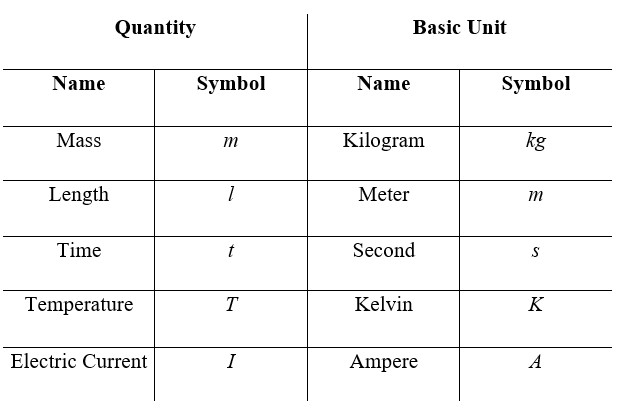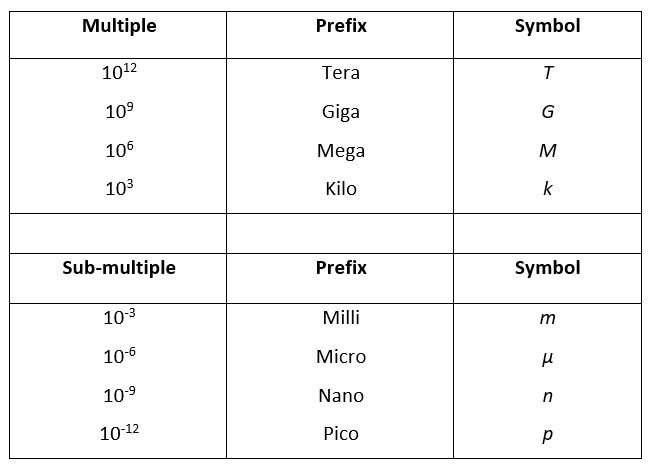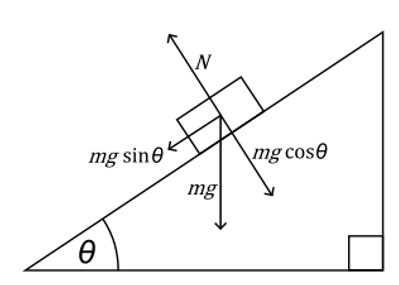4 Ijesha Close, Ilupeju, Lagos
+2347 086 296 002

#### Quantities, Mass, and Weight

##### QUANTITIES
• A physical quantity is made up of magnitude and unit.###### Base Units
• The following are base units:• All units (not from the table above) can be broken to base units.
• Homogeneity can be used prove equations.
• An equation is homogeneous if the base units on the left hand side are the same as the base units on the right hand side.
• This may not work all the time due to the fact that it does not take pure numbers into account.
###### Multiples and Sub-multiples###### Scalar and Vector
• A scalar has magnitude only, it cannot be -ve. E.g. Speed, energy, power, work, mass, distance, etc.
• A vector has magnitude and direction, it can be -ve. E.g. Displacement, acceleration, force, velocity, momentum, weight, electric field strength, etc.Vectors

###### Measurement TechniquesUsing a Cathode Ray Oscilloscope (C.R.O.)

1. A supply of peak value, 5.0 V, and of frequency 50 Hz, is connected to a c.r.o with time-base at 10 ms per division, and Y-gain at 5.0 V per division. Which trace is obtained?• The maximum value is 5.0 V; therefore eliminate A and B
• F = 1/T and T = Time-base × Divisions
• ∴ F = 1/Time-base × Divisions
• Divisions = 1/F × Time-base = 1/50 × 10 × 10-3 = 2
• The trace must have a period of 2 divisions and a height of 1 division, therefore D.

Systematic and Random Errors

• Systematic Error:
• Constant error in one direction; too big or too small
• Cannot be eliminated by repeating or averaging
• If systematic error is small, the measurement is accurate.
• Accuracy refers to the degree of agreement between a result of measurement and the true value of the quantity.
• Random Error:
• Random fluctuations or scatter about a true value
• Can be reduced by repeating and averaging
• When random errors are small, the measurement is precise
• Precision refers to the degree of agreement of repeated measurements of the same quantity (regardless of whether it is correct or not)Calculations Involving Errors

• For a quantity x = (2.0 ± 0.1) mm
• Absolute uncertainty = Δx = ± 0.1 mm
• Fractional uncertainty = Δx/x = 0.05
• Percentage uncertainty = Δx/x × 100% = 5%
• Combining errors:
• If p = 2x + y/3 or p = 2x – y/3, then Δp = 2Δx + Δy/3
• When the values are multiplied or divided, add percentage errors
• When values are powered (e.g. squared), multiply the percentage error with the power.
• If r = 2xy3 or r = 2x/y3, then Δr/r = Δx/x + 3Δy/y

Treatment of Significant Figures

• Actual error is recorded to only 1 significant figure.
• The number of decimal places for a calculated quantity is equal to the number of decimal places in the actual error.
• During a practical, when calculating using a measured quantity, give answers to the same significant figure as the measurement or one less.

Micrometer Screw Gauge• Measures objects up to 0.01 mm
• Place the object between the anvil and spindle
• Rotate thimble until the object is firmly held by the jaws.
• Add the values from the main scale and the rotating scale.

Vernier Scale• Measures objects up to 0.1 mm
• Place the object on the rule
• Push the slide scale to the edge of the object
• The sliding scale is 0.9 mm long and is divided into 10 equal divisions
• Check which line division on the sliding scale matches with a line division on the rule
• Subtract the value from the sliding scale (0.09 × divisions) by the value from the rule
##### MASS AND WEIGHT
• Mass is the amount of matter an object contains, and is a property that ‘resists’ change in motion.
• Weight is the force of gravity acting on an object, measured in Newtons, and given by the formula: Weight = Mass × Gravity
• Weights (and hence masses) may be compared using a balance.
Minimum 4 characters